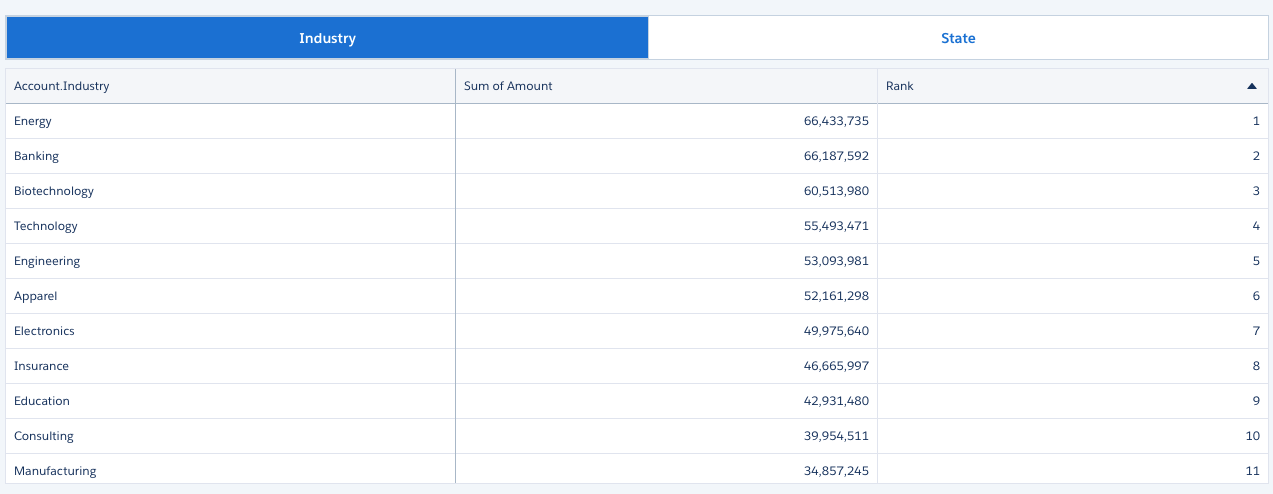Einstein Analytics: Demystifying Bindings – Part 6

08. April 2019 0

If you have been looking at some of my other blogs, you might know I love the compare table functionality, we get to do powerful calculations without writing code. Now I know that we of course also want our compare tables to be dynamic, so next in the binding agenda is compare table bindings.

Compare Table Group Binding

As always we need to do some prep work and in this case we need to have two things; the static step and the compare table step.

If we look at the static step I am planning a simple group selection allowing the user to switch between ‘Industry’ and ‘Billing State’. The step looks like this:

```"values": [
{
"display": "Industry",
"value": "Account.Industry"
},
{
"display": "State",
"value": "Account.BillingState"
}
]```

The compare table is pretty simple, it consists of the grouping ‘Industry’ (of course) and ‘sum of Amount’. To leverage the compare table functionality I did a simple rank within group function. The query looks like this

```"query": {
"columns": [
{
"query": {
"measures": [
[
"sum",
"Amount"
]
],
"groups": [
"Account.Industry"
]
}
},
{
"query": {
"measures": [
[
"sum",
"Amount"
]
],
"groups": [
"Account.Industry"
],
"formula": "rank() over([..] partition by all order by A desc)",
"order": [
[
-1,
{
"ascending": true,
"inner": true
}
]
]
},
}
],
"measures": [
[
"sum",
"Amount",
"A"
],
[
"sum",
"Amount",
"B"
]
],
"groups": [
"Account.Industry"
]
}```

Now first of all your JSON might have a different order than me, that is alright. A compare table has different components and these can come in any order. We will, of course, look at these components in the next section.

The dashboard before the binding has been applied looks like this:The Binding in Details

Looking at the JSON for the compare table you will notice that the query has three main components: “measures”, “groups” and “columns”. The measures section contain the two measures we added which in my case is two sum of Amount. You will also notice that they have A and B which refers to the column alias you see in the UI. The groups just contains the group and finally, we have columns. The column section is a little bit more complex, this is where we have the query for each column (measure added in the UI). For each of these, we will see a grouping and a measure plus possibly a few more parameters like the formula for our rank function.

With this in mind, it is not enough with a single binding, we need to change all the references to the grouping. In the sample above that will be three bindings, which you can see highlighted below.

```"query": {
"columns": [
{
"query": {
"measures": [
[
"sum",
"Amount"
]
],
"groups": [
"Account.Industry"
]
}
},
{
"query": {
"measures": [
[
"sum",
"Amount"
]
],
"groups": [
"Account.Industry"
],
"formula": "rank() over([..] partition by all order by A desc)",
"order": [
[
-1,
{
"ascending": true,
"inner": true
}
]
]
},
}
],
"measures": [
[
"sum",
"Amount",
"A"
],
[
"sum",
"Amount",
"B"
]
],
"groups": [
"Account.Industry"
]
}```

The binding we have to use is pretty simple, it’s a column data selection referencing the static step and .asObject() for the data serialization.

`"{{column(Static_1.selection, [\"value\"]).asObject()}}"`

I can recommend going through the first part of this blog series if you need the binding described further.

In order to make the compare table dynamic we, of course, need to replace the three groupings with the binding like below.

```"query": {
"columns": [
{
"query": {
"measures": [
[
"sum",
"Amount"
]
],
"groups": "{{column(Static_1.selection, [\"value\"]).asObject()}}"
}
},
{
"query": {
"measures": [
[
"sum",
"Amount"
]
],
"groups": "{{column(Static_1.selection, [\"value\"]).asObject()}}",
"formula": "rank() over([..] partition by all order by A desc)",
"order": [
[
-1,
{
"ascending": true,
"inner": true
}
]
]
},
}
],
"measures": [
[
"sum",
"Amount",
"A"
],
[
"sum",
"Amount",
"B"
]
],
"groups": "{{column(Static_1.selection, [\"value\"]).asObject()}}"
}```

And that’s all it takes. The binding is the same as we have learned, we just need to change all the references to the grouping.Other Compare Table Bindings

If you have followed this blog series then you know that it’s not only groupings we can make dynamic. So if you want to make the measure or filter dynamic, that is possible as well. Just remember you need to replace all the references in the query with the binding.

In the next part, we will be looking at how to make bindings in values tables.

This site uses Akismet to reduce spam. Learn how your comment data is processed.